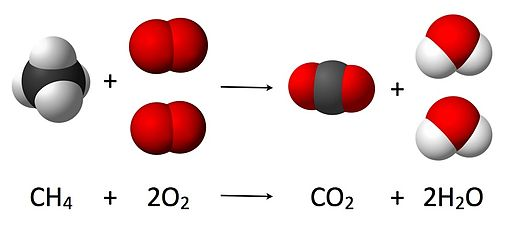10 minutes maximum! Can you do it in 5?1. Bond breaking ...
• A. needs energy and is an exothermic process
• B. needs energy and is an endothermic process
• C. produces energy and is an exothermic process
• D. produces energy and is an endothermic process
2. Bond making is ...
• A. exothermic which means that energy is required when bonds are made.
• B. exothermic which means that energy is released when bonds are made.
• C. endothermic which means that energy is required when bonds are made.
• D. endothermic which means that energy is released when bonds are made.
3. In an endothermic reaction, energy is ...
• A. released to the surroundings and the enthalpy (energy) is given a positive sign.
• B. released to the surroundings and the enthalpy (energy) is given a negative sign.
• C. absorbed from the surroundings and the enthalpy (energy) is given a positive sign.
• D. absorbed from the surroundings and the enthalpy (energy) is given a negative sign.
4. In an exothermic reaction, the energy needed to break existing bonds is ...
• A. greater than the energy needed to form the new bonds
• B. less than the energy needed to form the new bonds
• C. greater than the energy released on forming the new bonds.
• D. less than the energy released on forming the new bonds
5. Hydrogen chloride gas is produced by reacting hydrogen with chlorine. The equation for this reaction is:

H2  +   Cl2      2HCl

The energy needed to break the bonds in H2 and Cl2 is 679kJ and the energy released on the formation of the bonds in 2HCl is 856kJ.

The overall energy change in kilojoules for this reaction is:

• A.   +177
• B.   -177
• C.   +1535
• D.   -1535

Q6-8. When hydrogen ‘pops’ with a lighted splint it is reacting with oxygen to produce water. The equation for the reaction is:

2H2   +   O2      2H2O

 Bond Type Bond Energy  (kJ/mol) H-H 436 O=O 498 O-H 464

6. Calculate the energy required to break all the reactant molecules into individual atoms.
• A.  928kJ
• B.  934kJ
• C.  1370kJ
• D.  1856kJ

7. Calculate the energy released when the bonds in the product molecules are formed.

• A.  928kJ
• B.  934kJ
• C.  1370kJ
• D.  1856kJ

8. Calculate the overall enthalpy change (energy change) for the reaction.

• A. -486kJ
• B. +486kJ
• C. -922kJ
• D. +922kJ

Q9-10. The combustion of methane is illustrated below in both equation and model form:The relevant bond energy values are:

JyntoRobert
A. RohdeJacek
FHJynto

CC-BY-SA 3.0

 Bond type Bond energy (kJ/mol) C-H 415 O=O 498
 Bond type Bond energy (kJ/mol) C=O 799 O-H 464

9. The number of bonds being broken in the reactants and formed in the products is:

 Bonds broken Bonds formed C-H O=O C=O O-H A. 4 2 2 4 B. 1 2 1 2 C. 4 2 1 2 D. 1 2 2 4

10. The enthalpy (energy) change for this reaction is:

• A.  -350kJ
• B.  +350kJ
• C.  -798kJ
• D.  +798kJ

Image by Gerd Altmann from Pixabay